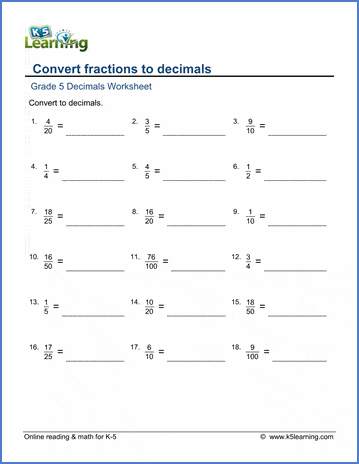# Converting Fractions and Decimals Worksheets

## Fractions, decimals & mixed numbers

These grade 5 math worksheets give students practice in converting between fractions, decimals and mixed numbers.Sample Grade 5 Fractions to / from Decimals Worksheet

## More decimals worksheets

Find all of our decimals worksheets, from converting fractions to decimals to long division of multi-digit decimal numbers.

## More fractions worksheets

Explore all of our fractions worksheets, from dividing shapes into "equal parts" to multiplying and dividing improper fractions and mixed numbers.

What is K5?

K5 Learning offers free worksheets, flashcards and inexpensive workbooks for kids in kindergarten to grade 5. Become a member to access additional content and skip ads.